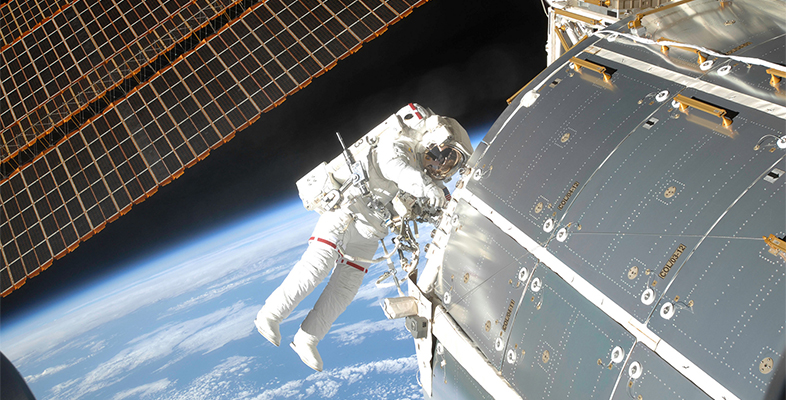Science, Maths & Technology

### Become an OU studentMicrogravity: living on the International Space Station

Start this free course now. Just create an account and sign in. Enrol and complete the course for a free statement of participation or digital badge if available.

# 4 How much do space missions cost the world?

How much do the space missions cost?

In Figure 4, the missions from Voyager 1 to Dawn are compared with the historical deployment of US troops in Iraq. This is the global cost of space missions after all so these global costs can be compared with the US deployment to Iraq as a direct comparison. Use this information to complete Activity 4.Figure 4 Relative costs per space mission.

## Activity 4 The cost of space missions

Timing: Allow approximately 10 minutes

Choose the correct answer to the following questions.

1. What is the total cost of all of the space missions shown in Figure 4?

a.

US$10.3 billion b. US$900 billion

c.

US$2500 billion d. US$3600 billion

e.

US$10 300 billion The correct answer is a. 2. Given that there are about 8 billion people on Earth, how much would this cost each person? (Hint: round your final answer to 2 significant figures.) a. US$1.30

b.

US$1.40 c. US$13.0

d.

US$14.0 e. US$130

3. As a proportion of one month’s deployment of US troops to Iraq, how much did the Rosetta mission cost?

a.

0.08%

b.

8%

c.

18%

d.

28%

e.

80%